Transplantation

Next: Gallery of examples Up: Some planar isospectral domains Previous: Introduction

# Transplantation

The following transplantation proof was first applied to Riemann surfaces by Buser . For our domains this proof turns out to be particularly easy.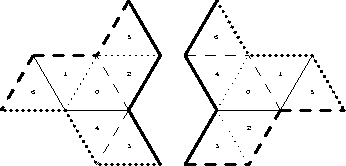Figure 2: Propeller example.

Consider the two propeller-shaped regions shown in Figure 2. Each region consists of seven equilateral triangles (labelled in some unspecified way). Our first pair of examples is obtained from these by replacing the equilateral triangles by acute-angled scalene triangles, all congruent to each other. The propellers are triangulated by these triangles in such a way that any two triangles that meet along a line are mirror images in that line, as in Figure 3. In both propellers the central triangle has a distinguishing property: its sides connect the three inward corners of the propeller. The position of the propellers in Figure 3 is such that the unique isometry from the central triangle on the left-hand side to the central triangle on the right-hand side is a translation. This translation does not map the propellers onto one another and so they are not isometric.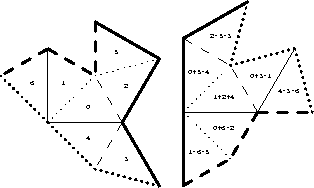Figure 3: Warped propeller.

Now let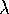be any real number, and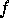any eigenfunction of the Laplacian with eigenvaluefor the Dirichlet problem corresponding to the left-hand propeller. Let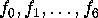denote the functions obtained by restrictingto each of the 7 triangles of the left-hand propeller, as indicated on the left in Figure 3. For brevity, we write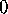for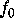,for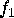, etc. The Dirichlet boundary condition is thatmust vanish on each boundary-segment. Using the reflection principle, this is equivalent to the assertion thatwould go into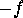if continued as a smooth eigenfunction across any boundary-segment. (More precisely it goes intowhere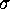is the reflection on the boundary segment.)

On the right in Figure 3, we show how to obtain fromanother eigenfunction of eigenvalue, this time for the right-hand propeller. In the central triangle, we put the function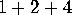. By this we mean the function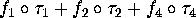where for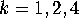,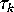is the isometry from the central triangle of the right-hand propeller to the triangle labelled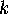on the left-hand propeller. Now we see from the left-hand side that the functions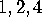continue smoothly across dotted lines into copies of the functions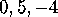respectively, so that their sum continues into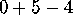as shown. The reader should check in a similar way that this continues across a solid line to(its negative), and across a dashed line to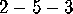, which continues across either a solid or dotted line to its own negative. These assertions, together with the similar ones obtained by symmetry (i.e. cyclic permutation of the arms of the propellers), are enough to show that the transplanted function is an eigenfunction of eigenvaluethat vanishes along each boundary segment of the right-hand propeller.

So we have defined a linear map which for eachtakes the-eigenspace for the left-hand propeller to the-eigenspace for the right-hand one. This is easily checked to be a non-singular map, and so the dimension of the eigenspace on the right-hand side is larger or equal the dimension on the left-hand side. Since the same transplantation may also be applied in the reversed direction the dimensions are equal. This holds for each, and so the two propellers are Dirichlet isospectral.

In fact they are also Neumann isospectral, as can be seen by a similar transplantation proof obtained by replacing every minus sign in the above by a plus sign. (Going from Neumann to Dirichlet is almost as easy: Just color the triangles on each side alternately black and white, and attach minus signs on the right to function elements that have moved from black to white or vice versa.)

In the propeller example, each of the seven function elements on the left got transplanted into three triangles on the right, and we verified that it all fits together seamlessly. If we hadn't been given the transplantation rule, we could have worked it out as follows: We start by transplanting the function elementinto the central triangle on the right; on the leftcontinues across a dotted line to, so we stickin the triangle across the dotted line on the right; on the leftcontinues across the solid line to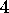, and since on the right the solid side of the triangle containingis a boundary edge, we stick ain along with the(don't worry about signs-we can fill them in afterwards using the black and white coloring of the triangles); now since on the leftcontinues across a dotted line to itself we stick ainto the center along with thewe started with; and so on until we have three function elements in each triangle on the right and the whole thing fits together seamlessly.

If we had begun by puttinginto the central triangle on the right, rather than, then we would have ended up with four function elements in each triangle, namely, the complement in the setof the original three; This gives a second transplantation mapping. Call the original mapping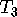, and the complementary mapping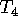. Any linear combination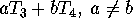will also be a transplantation mapping, and if we take forone of the four solutions to the equations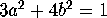,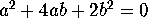, our transplantation mapping becomes norm-preserving.

Now consider the pair of putatively homophonic domains shown in Figure 1 above. In this case we find two complementary transplantation mappings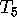and. The linear combination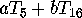is a norm-preserving mapping if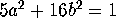and, that is, if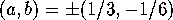or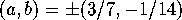. In the Dirichlet case, transplantation is kind to the values of the transplanted functions at the special interior points where six triangles meet. With the proper choice of sign, the Dirichlet incarnation ofmultiplies the special value by 2, the Dirichlet incarnation ofmultiplies the special value by, and the four norm-preserving linear combinationsspecified above multiply it by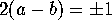. Thus we can convert an orthonormal basis of Dirichlet eigenfunctions on the left to one on the right so that corresponding functions take on the same special value. This shows that the two domains are homophonic, or more specifically, Dirichlet homophonic. There is no similar reason for these domains to be Neumann homophonic, and, in fact, we do not know of any pair of non-congruent Neumann homophonic domains.

Next: Gallery of examples Up: Some planar isospectral domains Previous: Introduction

Peter Doyle